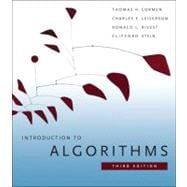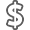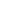# Introduction to Algorithms

• ISBN13:

• ISBN10:

## 0262033844

• Edition: 3rd
• Format: Hardcover
• Copyright: 2009-09-30
• Publisher: RANDOM HOUSE

Note: Supplemental materials are not guaranteed with Rental or Used book purchases.

Purchase Benefits

•Free Shipping On Orders Over \$35!
Your order must be \$35 or more to qualify for free economy shipping. Bulk sales, PO's, Marketplace items, eBooks and apparel do not qualify for this offer.
•Get Rewarded for Ordering Your Textbooks! Enroll Now
•We Buy This Book Back!
In-Store Credit: \$38.72
Check/Direct Deposit: \$36.88
PayPal: \$36.88
List Price: \$105.60 Save up to \$84.95
• Rent Book \$20.65Free Shipping

TERM
PRICE
DUE
IN STOCK USUALLY SHIPS IN 24 HOURS.
*This item is part of an exclusive publisher rental program and requires an additional convenience fee. This fee will be reflected in the shopping cart.

### Supplemental Materials

What is included with this book?

• The New copy of this book will include any supplemental materials advertised. Please check the title of the book to determine if it should include any access cards, study guides, lab manuals, CDs, etc.
• The Used and Rental copies of this book are not guaranteed to include any supplemental materials. Typically, only the book itself is included. This is true even if the title states it includes any access cards, study guides, lab manuals, CDs, etc.

### Summary

Some books on algorithms are rigorous but incomplete; others cover masses of material but lack rigor. Introduction to Algorithms uniquely combines rigor and comprehensiveness. The book covers a broad range of algorithms in depth, yet makes their design and analysis accessible to all levels of readers. Each chapter is relatively self-contained and can be used as a unit of study. The algorithms are described in English and in a pseudocode designed to be readable by anyone who has done a little programming. The explanations have been kept elementary without sacrificing depth of coverage or mathematical rigor. The first edition became a widely used text in universities worldwide as well as the standard reference for professionals. The second edition featured new chapters on the role of algorithms, probabilistic analysis and randomized algorithms, and linear programming. The third edition has been revised and updated throughout. It includes two completely new chapters, on van Emde Boas trees and multithreaded algorithms, substantial additions to the chapter on recurrence (now called "Divide-and-Conquer"), and an appendix on matrices. It features improved treatment of dynamic programming and greedy algorithms and a new notion of edge-based flow in the material on flow networks. Many new exercises and problems have been added for this edition. As of the third edition, this textbook is published exclusively by the MIT Press.

### Author Biography

Thomas H. Cormen is Professor of Computer Science and former Director of the Institute for Writing and Rhetoric at Dartmouth College. He is the coauthor (with Charles E. Leiserson, Ronald L. Rivest, and Clifford Stein) of the leading textbook on computer algorithms, Introduction to Algorithms (third edition, MIT Press, 2009).

Charles E. Leiserson is Professor of Computer Science and Engineering at the Massachusetts Institute of Technology.

Ronald L. Rivest is Andrew and Erna Viterbi Professor of Electrical Engineering and Computer Science at the Massachusetts Institute of Technology.

Clifford Stein is Professor of Industrial Engineering and Operations Research at Columbia University.

### Table of Contents

 Preface p. xiii Foundations Introduction p. 3 The Role of Algorithms in Computing p. 5 Algorithms p. 5 Algorithms as a technology p. 11 Getting Started p. 16 Insertion sort p. 16 Analyzing algorithms p. 23 Designing algorithms p. 29 Growth of Functions p. 43 Asymptotic notation p. 43 Standard notations and common functions p. 53 Divide-and-Conquer p. 65 The maximum-subarray problem p. 68 Strassen's algorithm for matrix multiplication p. 75 The substitution method for solving recurrences p. 83 The recursion-tree method for solving recurrences p. 88 The master method for solving recurrences p. 93 Proof of the master theorem p. 97 Probabilistic Analysis and Randomized Algorithms p. 114 The hiring problem p. 114 Indicator random variables p. 118 Randomized algorithms p. 122 Probabilistic analysis and further uses of indicator random variables p. 130 Sorting and Order Statistics Introduction p. 147 Heapsort p. 151 Heaps p. 151 Maintaining the heap property p. 154 Building a heap p. 156 The heapsort algorithm p. 159 Priority queues p. 162 Quicksort p. 170 Description of quicksort p. 170 Performance of quicksort p. 174 A randomized version of quicksort p. 179 Analysis of quicksort p. 180 Sorting in Linear Time p. 191 Lower bounds for sorting p. 191 Counting sort p. 194 Radix sort p. 197 Bucket sort p. 200 Medians and Order Statistics p. 213 Minimum and maximum p. 214 Selection in expected linear time p. 215 Selection in worst-case linear time p. 220 Data Structures Introduction p. 229 Elementary Data Structures p. 232 Stacks and queues p. 232 Linked lists p. 236 Implementing pointers and objects p. 241 Representing rooted trees p. 246 Hash Tables p. 253 Direct-address tables p. 254 Hash tables p. 256 Hash functions p. 262 Open addressing p. 269 Perfect hashing p. 277 Binary Search Trees p. 286 What is a binary search tree? p. 286 Querying a binary search tree p. 289 Insertion and deletion p. 294 Randomly built binary search trees p. 299 Red-Black Trees p. 308 Properties of red-black trees p. 308 Rotations p. 312 Insertion p. 315 Deletion p. 323 Augmenting Data Structures p. 339 Dynamic order statistics p. 339 How to augment a data structure p. 345 Interval trees p. 348 Advanced Design and Analysis Techniques Introduction p. 357 Dynamic Programming p. 359 Rod cutting p. 360 Matrix-chain multiplication p. 370 Elements of dynamic programming p. 378 Longest common subsequence p. 390 Optimal binary search trees p. 397 Greedy Algorithms p. 414 An activity-selection problem p. 415 Elements of the greedy strategy p. 423 Huffman codes p. 428 Matroids and greedy methods p. 437 A task-scheduling problem as a matroid p. 443 Amortized Analysis p. 451 Aggregate analysis p. 452 The accounting method p. 456 The potential method p. 459 Dynamic tables p. 463 Advanced Data Structures Introduction p. 481 B-Trees p. 484 Definition of B-trees p. 488 Basic operations on B-trees p. 491 Deleting a key from a B-tree p. 499 Fibonacci Heaps p. 505 Structure of Fibonacci heaps p. 507 Mergeable-heap operations p. 510 Decreasing a key and deleting a node p. 518 Bounding the maximum degree p. 523 van Emde Boas Trees p. 531 Preliminary approaches p. 532 A recursive structure p. 536 The van Emde Boas tree p. 545 Data Structures for Disjoint Sets p. 561 Disjoint-set operations p. 561 Linked-list representation of disjoint sets p. 564 Disjoint-set forests p. 568 Analysis of union by rank with path compression p. 573 Graph Algorithms Introduction p. 587 Elementary Graph Algorithms p. 589 Representations of graphs p. 589 Breadth-first search p. 594 Depth-first search p. 603 Topological sort p. 612 Strongly connected components p. 615 Minimum Spanning Trees p. 624 Growing a minimum spanning tree p. 625 The algorithms of Kruskal and Prim p. 631 Single-Source Shortest Paths p. 643 The Bellman-Ford algorithm p. 651 Single-source shortest paths in directed acyclic graphs p. 655 Dijkstra's algorithm p. 658 Difference constraints and shortest paths p. 664 Proofs of shortest-paths properties p. 671 All-Pairs Shortest Paths p. 684 Shortest paths and matrix multiplication p. 686 The Floyd-Warshall algorithm p. 693 Johnson's algorithm for sparse graphs p. 700 Maximum Flow p. 708 Flow networks p. 709 The Ford-Fulkerson method p. 714 Maximum bipartite matching p. 732 Push-relabel algorithms p. 736 The relabel-to-front algorithm p. 748 Selected Topics Introduction p. 769 Multithreaded Algorithms p. 772 The basics of dynamic multithreading p. 774 Multithreaded matrix multiplication p. 792 Multithreaded merge sort p. 797 Matrix Operations p. 813 Solving systems of linear equations p. 813 Inverting matrices p. 827 Symmetric positive-definite matrices and least-squares approximation p. 832 Linear Programming p. 843 Standard and slack forms p. 850 Formulating problems as linear programs p. 859 The simplex algorithm p. 864 Duality p. 879 The initial basic feasible solution p. 886 Polynomials and the FFT p. 898 Representing polynomials p. 900 The DFT and FFT p. 906 Efficient FFT implementations p. 915 Number-Theoretic Algorithms p. 926 Elementary number-theoretic notions p. 927 Greatest common divisor p. 933 Modular arithmetic p. 939 Solving modular linear equations p. 946 The Chinese remainder theorem p. 950 Powers of an element p. 954 The RSA public-key cryptosystem p. 958 Primality testing p. 965 Integer factorization p. 975 String Matching p. 985 The naive string-matching algorithm p. 988 The Rabin-Karp algorithm p. 990 String matching with finite automata p. 995 The Knuth-Morris-Pratt algorithm p. 1002 Computational Geometry p. 1014 Line-segment properties p. 1015 Determining whether any pair of segments intersects p. 1021 Finding the convex hull p. 1029 Finding the closest pair of points p. 1039 NP-Completeness p. 1048 Polynomial time p. 1053 Polynomial-time verification p. 1061 NP-completeness and reducibility p. 1067 NP-completeness proofs p. 1078 NP-complete problems p. 1086 Approximation Algorithms p. 1106 The vertex-cover problem p. 1108 The traveling-salesman problem p. 1111 The set-covering problem p. 1117 Randomization and linear programming p. 1123 The subset-sum problem p. 1128 Appendix: Mathematical Background Introduction p. 1143 Summations p. 1145 Summation formulas and properties p. 1145 Bounding summations p. 1149 Sets, Etc. p. 1158 Sets p. 1158 Relations p. 1163 Functions p. 1166 Graphs p. 1168 Trees p. 1173 Counting and Probability p. 1183 Counting p. 1183 Probability p. 1189 Discrete random variables p. 1196 The geometric and binomial distributions p. 1201 The tails of the binomial distribution p. 1208 Matrices p. 1217 Matrices and matrix operations p. 1217 Basic matrix properties p. 1222 Bibliography p. 1231 Index p. 1251 Table of Contents provided by Publisher. All Rights Reserved.